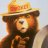# Convert smoothed moving average to ToS

P

#### Piper2808t

##### Member
2019 Donor
VIP
Can this be created in tos. its from trading view

Code:
``````study(title="Smoothed Moving Average", shorttitle="SMMA", overlay=true, resolution="")
len = input(7, minval=1, title="Length")
src = input(close, title="Source")
smma = 0.0
smma := na(smma) ? sma(src, len) : (smma * (len - 1) + src) / len
plot(smma, color=color.red)``````

M

#### metaller

##### New member
VIP
Code:
``````# Smoothed Moving Average
# Converted to thinkorswim from trading view by Alex Ivanov aka Metaller
# Sep 21, 2020

input len = 7;
input src = close;

rec smma  = if (IsNaN(smma) or smma==0) then SimpleMovingAvg(src, len) else (smma * (len - 1) + src) / len;

plot smma_plot = smma;
smma_plot.setDefaultColor(color.red);``````

•BenTen
M

#### metaller

##### New member
VIP
Interesting enough that SMMA provides virtually identical output as Wilders Moving Average :

Code:
``````input AvgType = AverageType.WILDERS;
input Price = close;
input Length = 7;
plot Data = MovingAverage(AvgType, Price, Length);``````

There is very small difference for first about 14 bars of chart (when len =7).How do I convert this ATR% chart study into a scanner watchlist column? Questions 5